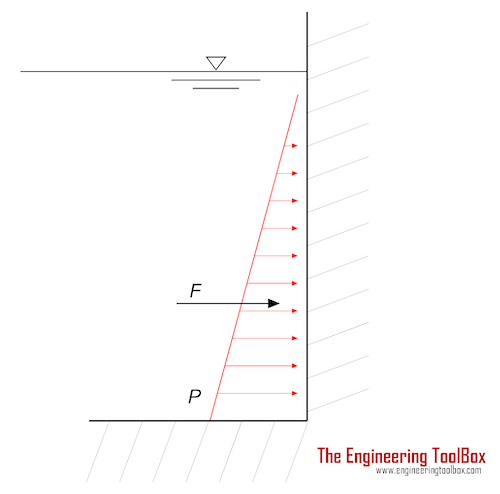Engineering ToolBox - Resources, Tools and Basic Information for Engineering and Design of Technical Applications!

# Hydrostatic Force acting on Submerged Surface

## Calculate the thrust force acting on a submerged surface.The thrust force acting on a surface submerged in a liquid can be calculated as

F = pa

=ρ g ha A                           (1)

where

F = thrust force (N)

pa = ρ g ha = average pressure on the surface (Pa)

A = area of submerged surface (m2)

ha = average depth (m)

ρ = density (kg/m3)  (water 1000 kg/m3)

g = acceleration of gravity (9.81 m/s2

### Example - The thrust force acting on the side of a container

The trust force acting on the submerged vertical side of a container can be calculated as

F = pa

= ((pt + pb) / 2) A

= (ρ g (ht + hb) / 2) A                         (2)

where

pt = pressure at the top of the submerged surface (Pa)

pb = pressure at the bottom of the submerged surface (Pa)

ht = depth at the top of the submerged surface (m)

hb = depth at the bottom of the submerged surface (m)

The thrust on a surface with width 1 m and height from 0 m to 2 m - in a water-filled container can be calculated as

F =  (ρ g (ht + hb) / 2) A

= (1000 kg/m3) (9.81 m/s2) ((0 m) + (2 m)) / 2) ((1 m) (2 m))

= 19620 N

= 19.6 kN

### Example - The thrust force acting on the bottom of a container

The trust force acting on the horizontal bottom of a submerged container can be calculated as

F =  pb A

= ρ g hb A                                     (2b)

The thrust on a bottom with width 1 m and length 2 m - on depth 1 m - in a water filled container can be calculated as

F =  ρ g hb A

= (1000 kg/m3) (9.81 m/s2) (1 m) ((1 m) (2 m))

= 19620 N

= 19.6 kN

## Related Topics

• Fluid Mechanics - The study of fluids - liquids and gases. Involving velocity, pressure, density and temperature as functions of space and time.

## Engineering ToolBox - SketchUp Extension - Online 3D modeling!

Add standard and customized parametric components - like flange beams, lumbers, piping, stairs and more - to your Sketchup model with the Engineering ToolBox - SketchUp Extension - enabled for use with the amazing, fun and free SketchUp Make and SketchUp Pro .Add the Engineering ToolBox extension to your SketchUp from the SketchUp Pro Sketchup Extension Warehouse!

Translate

## Privacy

We don't collect information from our users. Only emails and answers are saved in our archive. Cookies are only used in the browser to improve user experience.

Some of our calculators and applications let you save application data to your local computer. These applications will - due to browser restrictions - send data between your browser and our server. We don't save this data.

## Citation

• Engineering ToolBox, (2011). Hydrostatic Force acting on Submerged Surface. [online] Available at: https://www.engineeringtoolbox.com/thrust-submerged-surface-d_1767.html [Accessed Day Mo. Year].

Modify access date.

. .

#### Scientific Online Calculator6 24